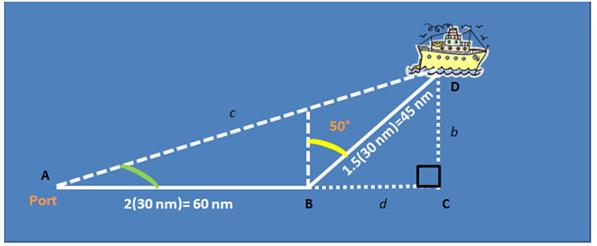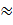### Sample Problem

A ship leaves the port at 8 AM and heads east at 30 nautical miles per hour.

At 10 A.M. the ship changes course to N 50° E (as shown in the diagram below).

Find the ship’s bearing and distance from the port of departure at 11:30 A.M.

Ship’s bearing: N ° E (round to the nearest whole degree)#### SolutionAngle DAB, or angle A (in green) will tell us the bearing of the ship.

For triangle BCD, B=90°-50°=40°. Sin 40°=b/45 → b=45sin 40° ; cos 40°=d/45 → d=45cos40°

Tan A=b/60+d=45sin40/(60+45cos40)0.30618

A=17.02°

The angle that angle A makes with the north-south line (bearings are measured in

degrees clockwise from north) is 90°-17.02°=72.98° → N 73° E

As for the distance c from the port to the ship,

Sin A=b/c=45sin40/c → c=45sin40/sin A=45sin40/sin(tan-1(45sin40/(60+45cos40)))98.8 nm

(distance from port)